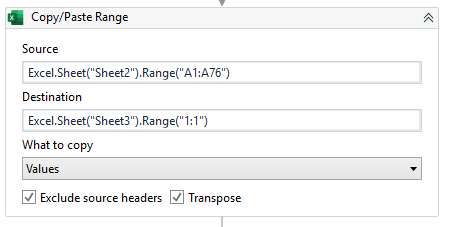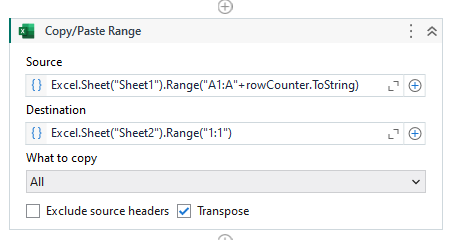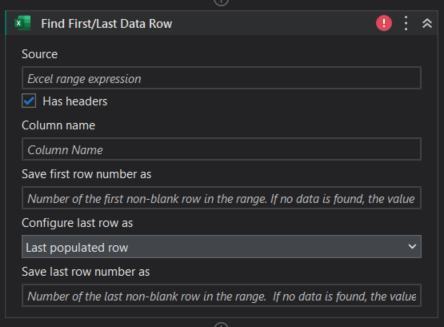# Use variable in the excel scope

Hi all,

I’m trying to copy a column and paste + transpose at the same time, when the source is:
Excel.Sheet(“Sheet2”).Range(“A:A”),

error would occur, so I tried a range here:
Excel.Sheet(“Sheet2”).Range(“A1:A76”)

The problem is the ‘76’ in this expression would change every time, so I would like to use a variable to replace it.

Wondering how should the variable be inserted in the expression below:
Excel.Sheet(“Sheet2”).Range(“A1:Avariable”)Thank you~

` Excel.Sheet("Sheet2").Range("A1:A" + Variabletoabeinserted)`

Cheers

1 Like

you can try as mentioned the belowhere rowCounter is an integer value which holds the row value of the last row.

1 Like

Hi @Sharath_HK ,

Can we use an activity in Studio to get the rowCounter? Cause when I tried to use in directly, it shows the variable is not declared. Thank you.

You could use the “Find First/Last Data Row” activity to get the last row in a column.1 Like

Hi @Anil_G ,

Thank you, it’s working with some followup adjustments.

1 Like

Hi @yikwen.goo ,